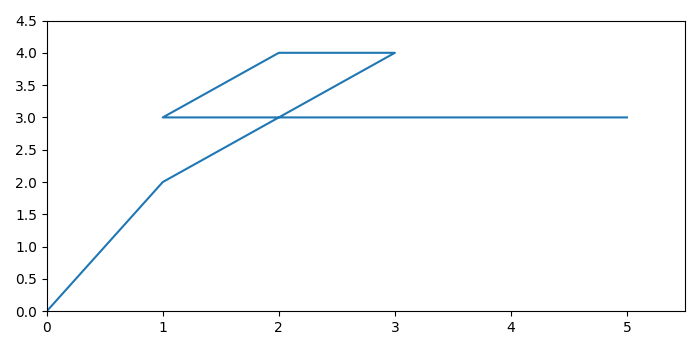# How to show (0,0) on matplotlib graph at the bottom left corner?

To show (0,0) on matplotlib graph at the bottom left corner, we can use xlim() and ylim() methods.

## Steps

• Set the figure size and adjust the padding between and around the subplots.

• Make lists of data points for x and y.

• Plotx and y data points.

• Setx and y axes scale.

• To display the figure, use Show() method.

## Example

import numpy as np
import matplotlib.pyplot as plt

plt.rcParams["figure.figsize"] = [7.00, 3.50]
plt.rcParams["figure.autolayout"] = True

x = np.array([0, 1, 3, 2, 1, 5])
y = np.array([0, 2, 4, 4, 3, 3])

plt.plot(x, y)

plt.xlim([0, max(x)+0.5])
plt.ylim([0, max(y)+0.5])

plt.show()



## Output

It will produce the following output −